X. PITFALLS IN DIGITIZING THE DATA

The advent and prolific use of digital computers has changed the manner in which processing of analog signals takes place if a computer is used. This section addresses the most common problems in such analyses.

10.1 Discrete-Continuous Processes

Digital processing implies that data must be presented to a computer or other processor as an array of numbers whether in a batch or in a time series. If the data are not already in this form (it usually is not when considering frequency stability measurements), then it is necessary to transform to this format by digitizing. Usually, the signal available for analysis is a voltage which varies with frequency or phase difference between two oscillators.

10.2 Digitizing the Data

Digitizing the data is the process of converting a continuous waveform into discrete numbers. The process is completed in real time using an analog-to-digital converter (ADC). Three considerations in the ADC are of importance here:

1.Conversion time

2.Resolution (quantization uncertainty)

3.Linearity

An ADC "looks at" an incoming waveform at equispaced intervals of time T. Ideally, the output of the ADC is the waveform (denoted by y(t)) multiplied by a series of infinitely narrow sampling intervals of unit height as in figure 10.1. We have at t = T

yl(t) = y(t)d (t-T) = y(T)d (t-T) (10.1)

where d (t-T) is a delta function. If y(t) is continuous at t = nT and n = 0, ±1, ±2,..., then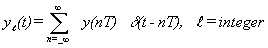(10.2)

The delta function representation of a sample waveform eq (10.2) is useful when a subsequent continuous integration is performed using it.6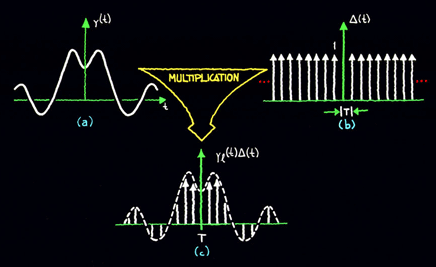Figure 10.1

In ADC's, the input signal is sampled during an aperture time and held for conversion to a digital number, usually binary. Sampling and processing takes time which is specified as the conversion time. This is the total time required for a complete measurement at one sample to achieve a given level of accuracy. If yl(t) is the ideal discrete-time representation of continuous process y(t), then the ADC output denoted by y'l(t) is: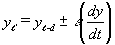(10.3)

where "d" is the conversion time and e × dy/dt the accuracy tolerance at "d" as a function of rate-of-change in y(t). In general a trade-off exists between d and e . For example, for a commonly available, high-quality 10-bit ADC, a conversion time of d = 10 m s yields a maximum error of 3%. Whereas given a 30 m s conversion time, we can obtain 0.1% maximum error.

The error due to conversion time "d" is many times negligible since processing in digital filters and spectrum analysis takes place after the converter. Conversion time delay can be of critical concern, however, where real-time processing at speeds of the order of "d" become important such as in digital servo loops where corrections are needed for fast changing errors.

A portion of the conversion-time error is a function of the rate of change dy/dt of the process if the sample-and-hold portion of the ADC relies on the charging of a capacitor during an aperture time. This is true because the charge cycle will have a finite time-constant and because of aperture time uncertainty. For example, if the time-constant is 0.1 ns (given by say a 0.1 W source resistance charging a 0.001 m fd capacitor), then a 0.1% nominal error will exist for slope D y/D t = 1V/m s due to charging. With good design, this error can be reduced. The sampling circuit (before charge) is usually the dominant source of error and logic gate-delay jitter creates an aperture time uncertainty. The jitter typically is between 2-5 ns which means an applied signal slewing at, say, 1 V/m s produces an uncertainty of 2-5 mV. Since e × dy/dt is directly proportional to signal slewing rate, it can be anticipated that high-level, high-frequency components of y(t) will have the greatest error in conversion. For typical ADC's, less than 0.1% error can be achieved by holding D y/D t to less than 0.2 V/m s.

The continuous process y(t) is partitioned into 2n discrete ranges for n-bit conversion. All analog values within a given range are represented by the same digital code, usually assigned to the nominal midrange value. There is, therefore, an inherent quantization uncertainty of ± ˝ least-significant bit (LSB) in addition to other conversion errors. For example, a 10-bit ADC has a total of 1024 discrete ranges with a lowest order bit then representing about 0.1% of full scale and quantization uncertainty of ± 0.05%.

We define the dynamic range of a digital system as the ratio between the maximum allowable value of the process (prior to any overflow condition) and the minimum discernable value. The dynamic range when digitizing the data is set by the quantizing uncertainty, or resolution, and is the ratio of 2n to ˝ LSB. (If additive noise makes coding ambiguous to the ˝ LSB level, then the dynamic range is the ratio of 2n to the noise uncertainty, but this is usually not the case.) For example, the dynamic range of a 10-bit system is 210 (= 1024) to ˝, or 2048 to 1. Expressed in dB's, this is

20 log 2048 = 66.2 dB

if referring to a voltage-to-code converter.

The converter linearity specifies the degree to which the voltage-to-code transfer approximates a straight line. The nonlinearity is the deviation from a straight line drawn between the end points (all zeros to all ones code). It is usually not acceptable to have nonlinearity greater than ˝ LSB which means that the sum of the positive errors or the sum of the negative errors of the individual bits must not exceed ˝ LSB (or ± ˝ LSB). The linearity specification used in this context includes all effects such as temperature errors under expected operating temperature extremes and power supply sensitivity errors under expected operating supply variations.

10.3 Aliasing

Figure 10.1 illustrates equispaced sampling of continuous process y(t). It is important to have a sufficient number of samples/second to properly describe information in the high frequencies. On the other hand, sampling at too high a rate may unnecessarily increase the processing labor. As we reduce the rate we see that sample values could represent low or high frequencies in y(t). This property is called aliasing and constitutes a source of error similar to "imaging" which occurs in analog frequency mixing schemes (i.e., in the multiplication of two different signals).

If the time between samples (k) is T seconds, then the sampling rate is 1/T samples per second. Then useful data in y(t) will be from 0 to 1/2T Hz and frequencies higher than 1/2T Hz will be "folded" into the lower range from 0 to 1/2T Hz and confused with data in this lower range. The cutoff frequency is then given by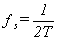(10.4)

We can use the convolution theorem to simply illustrate the existence of aliases. This theorem states that multiplication in the time domain corresponds to convolution in the frequency domain, and the time domain and frequency domain representations are Fourier transform pairs.7 The Fourier transform of y(t) in figure 10.1(a) is denoted by Y(f); thus: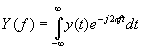(10.5) and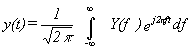(10.6)

The function Y(f) is depicted in figure 10.2(a). The Fourier transform of D (t) is shown in figure 10.2(b) and is given by D (f) where applying the discrete transform yields: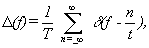(10.7) recalling that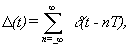(10.8)

from eq (10.2)

Y(f) convolved with D f is denoted by Y(f)* D (f) and is shown in figure 10.2(c). We see that the transform Y(f) is repeated with origins at f = n/T. Conversely, high frequency data with information around f = n/T will fold into the data around the origin between -fs and +fs. In the computation of power spectra, we encounter errors as shown in figure 10.3.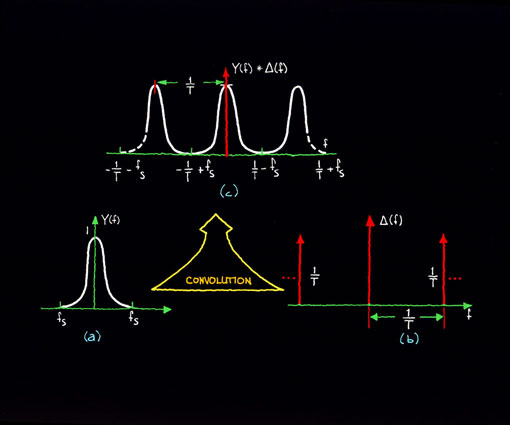Figure 10.2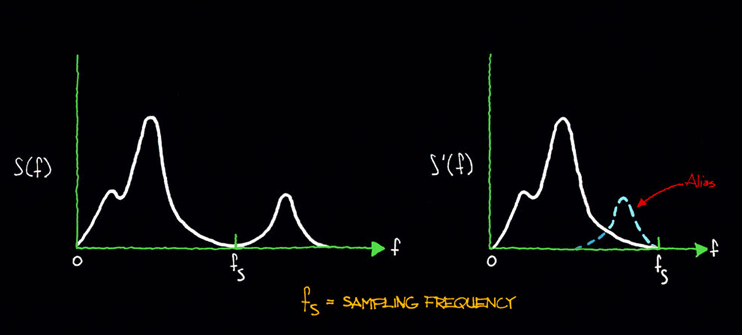Figure 10.3

Two pioneers in information theory, Harold Nyquist and Claude Shannon, developed design criteria for discrete-continuous processing systems. Given a specified accuracy, we can convey time-domain process y(t) through a finite bandwidth whose upper limit fN is the highest significant spectral component of y(t). For discrete-continuous process yk(t), ideally the input signal spectrum should not extend beyond fs, or

fN £ fs (10.9)

where fs is given by eq (10.4). Equation (10.9) is referred to as the "Nyquist limit."

In practice, there is never a case in which there is absolutely no signal or noise component above fN. Filters are used before the ADC in order to suppress components above fN which fold into the lower bandwidth of interest. This so-called anti-aliasing filter usually must be quite sophisticated in order to have low ripple in the passband, constant phase delay in the passband, and steep rolloff characteristics. In examining the rolloff requirements of the anti-aliasing filter, we can apply a fundamental filter property that the output spectrum is equal to the input spectrum multiplied by the square of the frequency response function; that is,

S(f) [H(f)]2 = Sout(f) (10.10)

The filter response must be flat to fN and attenuate aliased noise components at n/T ± f = 2nfs ± f. In digitizing the data, the observed spectra will be the sum of the baseband spectrum (to fN) and all spectra which are folded into the baseband spectrum

Sobserved(f) = S0(f) + S-1 (2fs - f) + S+1 (2fs + f)

 + S-2(4 fs - f).... + Si (2i (fs + f )) (10.11)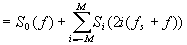where M is an appropriate finite limit.

For a given rejection at an upper frequency, clearly the cutoff frequency fc for the anti-aliasing filter should be as low as possible to relax the rolloff requirements. Recall that an nth order low-pass filter has frequency response function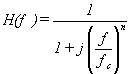(10.12) and output spectrum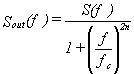(10.13) and after sampling, we have (applying eq (10.11))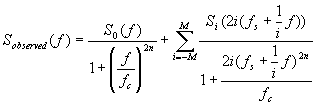(10.14)

If fc is chosen to be higher than fN, then the first term (baseband spectrum) is negligibly affected by the filter, which is our hope. It is the second term (the sum of the folded in spectra) which causes an error.

As an example of the rolloff requirement, consider the measurement of noise process n(t) at f = 400 Hz in a 1 Hz bandwidth on a digital spectrum analyzer. Suppose n(t) is white; that is,

Sn(f) = k0 (10.15)

k0 = constant

Suppose further that we wish to only measure the noise from 10 Hz to 1 kHz; Thus fN = 1 kHz. Let us assume a sampling frequency of fs = 2fN or 2 kHz. If we impose a 1 dB error limit in Sobserved and have 60 dB of dynamic range, then we can tolerate an error limit of 10-6 due to aliasing effects in this measurement, and the second term in eq (10.14) must be reduced to this level. We can choose fc = 1.5 kHz and obtain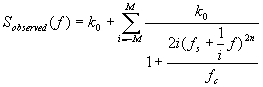(10.16)

The term in the series which contributes most is at i = -1, the nearest fold-in. The denominator must be 106 or more to realize the allowable error limit and at n ³ 8 this condition is met. The next most contributing term is i = +1 at which the error is < 10-7 for n = 8, a negligible contribution. The error increases as f increases for a fixed n because the nearest fold-in (i = -1) is coming down in frequency (note fig. 10.2(c)) and power there is filtered less by the anti-aliasing filter. Let us look at the worst case (f = 1 kHz) to determine a design criteria for this example. At f = 1 kHz, we must have n ³ 10.

Thus the requirement in this example is for a 10-pole low-pass filter (60 dB/octave rolloff).

10.4 Some History of Spectrum Analysis Leading to the Fast Fourier Transform

Newton in his Principia (1687) documented the first mathematical treatment of wave motion although the concept of harmonics in nature was pointed out by Pythagoras, Kepler, and Galileo. However, it was the work of Joseph Fourier in 1807 which showed that almost any function of a real variable could be represented as the sum of sines and cosines. The theory was rigorously treated in a document in 1822.

In using Fourier's technique, the periodic nature of a process or signal is analyzed. Fourier analysis assumes we can apply fixed amplitudes, frequencies and phases to the signal.

In the early 1900's two relatively independent developments took place: (1) radio electronics and electric power hardware were fast growing technologies; and (2) statistical analysis of events or processes which were not periodic became increasingly understood. The radio engineer explored signal and noise properties of a voltage or current into a load by means of the spectrum analyzer and measurement of the power spectrum. On the other hand, statisticians explored deterministic and stochastic properties of a process by means of the variance and self-correlation properties of the process at different times. Wiener (1930) showed that the variance spectrum (i.e., the breakdown of the variance with Fourier frequency) was the Fourier transform of the autocorrelation function of the process. He also theorized that the variance spectrum was the same as the power spectrum normalized to unit area. Tukey (1949) advocated the use of the variance spectrum in the statistical treatment of all processes because (1) it is more easily interpreted than correlation-type functions; and (2) it fortuitously is readily measurable by the radio engineer.

The 1950's saw rigorous application of statistics to communication theory. Parallel to this was the rapid advancement of digital computer hardware. Blackman and Tukey (1959) and Welch (1961) elaborated on other useful methods of deriving an estimate for the variance spectrum by taking the ensemble time-average sampled, discrete line spectra. The approach assumes the random process is ergodic. Some digital approaches estimate the variance spectrum using Wiener's theorem if correlation-type functions are useful in the analysis, but in general the time-averaged, sample spectrum is the approach taken since its implementation is direct and straightforward. Most always, ergodicity can be assumed.8,9

The variance of process y(t) is related to the total power spectrum by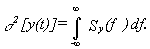(10.17) Since for zero mean,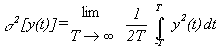(10.18)

we see that if y(t) is a voltage or current into a 1-ohm load, then the mean power of y(t) is the integral of Sy(f) with respect to frequency over the entire range of frequencies (-¥ ,¥ ). Sy(f) is, therefore, the power spectrum of process y(t). The power spectrum curve shows how the variance is distributed with frequency and should be expressed in units of watts per unit of frequency, or volts squared per unit of frequency when the load is not considered.

Direct estimation of power spectra has been carried out for many years through the use of analog instruments. These have variously been referred to as sweep spectrum analyzers, harmonic analyzers, filter banks, and wave analyzers. These devices make use of the fact that the spectrum of the output of a linear system (analog filter) is the spectrum of the input multiplied by the square of the system's frequency response function (real part of the transfer characteristic). Note eq (10.10). If y(t) has spectrum Sy(f) feeding a filter with frequency response function H(f), then its output is

Sfiltered(f) = [H(f)]2 Sy(f). (10.19)

If H(f) is rectangular in shape with width D f, then we can measure the contribution to the total power spectrum due to Sy(f ± D f/2).

Digital spectrum analysis is realized using the discrete Fourier transform (DFT), a modified version of the continuous transform depicted in eqs (10.5) and (10.6). By sampling the input waveform y(t) at discrete intervals of time tl = D t representing the sampled waveform by eq (10.2) and integrating eq (10.5) yields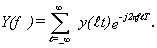(10.20)

Equation (10.20) is a Fourier series expansion. Because f(t) is specified as being bandlimited, the Fourier transform as calculated by eq (10.20) is as accurate as eq (10.5); however, it cannot extend beyond the Nyquist frequency, eq (10.4).

In practice we cannot compute the Fourier transform to an infinite extent, and we are restricted to some observation time T consisting of n D t intervals. This produces a spectrum which is not continuous in f but rather is computed with resolution D f where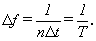(10.21) With this change, we get the discrete finite transform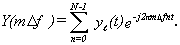(10.22)

The DFT computes a sampled Fourier series, and eq (10.22) assumes that the function y(t) repeats itself with period T. Y(mD f) is called the "line spectrum." A comparison of the DFT with the continuous Fourier transform is shown later in part 10.7.

We must calculate both the magnitude and phase of a frequency in the line spectrum, i.e., the real and imaginary part at a given frequency. N points in the time domain allow N/2 complex quantities in the frequency domain.

The power spectrum of y(t) is computed by squaring the real and imaginary components, adding the two together, and dividing by the total time T. We have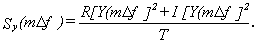(10.24)

This quantity is the sampled power spectrum and again assumes periodicity in process y(t) with total period T.10

10.5 Leakage

Sampled digital spectrum analysis always involves transforming a finite block of data. Continuous process y(t) is "looked at" for T time through a data window which can functionally be described by

y'(t) = w(t)× y(t)     (10.25)

where w(t) is the time domain window. The time-discrete counterpart to eq (10.25) is

yl'(t) = wl(t)× yl(t)     (10.26)

and wl(t) is now the sampled version of w(t) derived similarly to eq (10.2). Equation (10.26) is equivalent to convolution in the frequency domain, or

Y'(mD f) = W(mD f)*Y(mD f)     (10.27)

Y'(mD f) is called the "modified" line spectrum due to convolution of the original line spectrum with the Fourier transform of the time-domain window function.

Suppose the window function is rectangular, and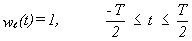(10.28)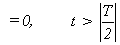This window is shown in figure 10.4(a). The Fourier transform of this window is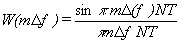(10.29)

and is shown in figure 10.4(b).

If y(t) is, for example, a sine wave, we convolve the spectrum of the sinusoid, a delta function, with W(mD f) to get the spectrum which is actually observed. Another way of describing this effect is to say that the transform process (eq 10.22) treats the sample signal as if it were periodically extended. Discontinuities usually occur at the ends of the window function in the extended version of the sampled waveform as in figure 10.5(c). Sample spectra thus represent a periodically extended sampled waveform, complete with discontinuities at its ends, rather than the original waveform.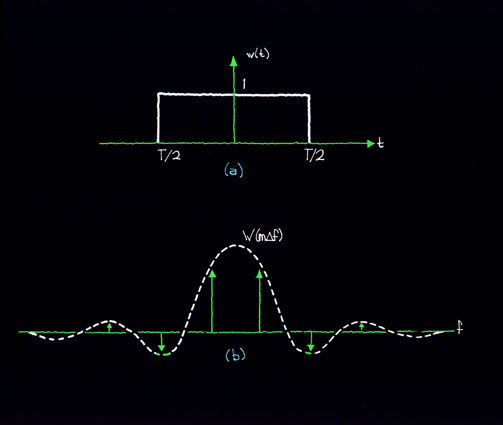Figure 10.4

Spurious components appear near the sinusoid spectrum and this is referred to as "leakage." Leakage results from discontinuities in the periodically extended sample waveform.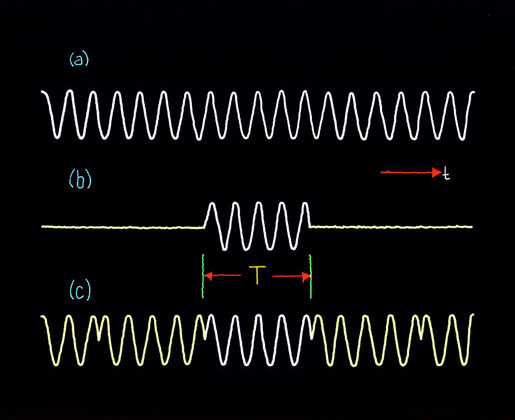Figure 10.5

Leakage cannot be eliminated entirely, but one can choose an appropriate window function w(t) in order to minimize its effect. This is usually done at the expense of resolution in the frequency domain. An optimum window for most cases is the Hanning window given by: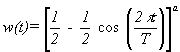(10.30)

for 0 £ t £ T and "a" designates the number of times the window is implemented. Figure 10.6(b) shows the Hanning line shape in the frequency domain for various numbers of "Hanns." Note that this window eliminates discontinuities due to the ends of sample length T.

Each time the Hanning window is applied, the sidelobes in the transform are attenuated by 12 dB/octave, and the main lobe is widened by 2D f. The amplitude uncertainty of an arbitrary sine wave input is reduced as we increase the number of Hanns; however, we trade off resolution in frequency.

The effective noise bandwidth is the difference of the filter response away from a true rectangularly shaped filtered response (frequency domain). Table 10.1 lists equivalent noise bandwidth corrections for up to three applications of the Hanning window.11

TABLE 10.1
 Number of Hanns Equivalent Noise Bandwidth 1 1.5 Df 2 1.92 Df 3 2.31 Df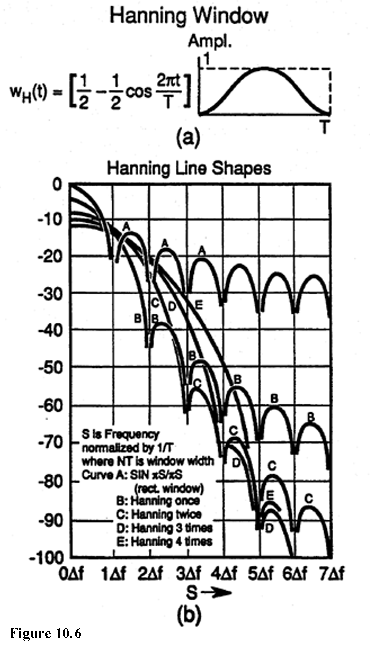Main Page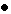Table of ContentsGo to section:

Summary and IntroductionIIIIIIIVVVIVIIVIIIIXXXIXIIConclusionReferences